ROOT   Reference GuideAdvanced 2-dimensional spectra processing.

This class contains advanced spectra processing functions.

• One-dimensional background estimation functions
• Two-dimensional background estimation functions
• One-dimensional smoothing functions
• Two-dimensional smoothing functions
• One-dimensional deconvolution functions
• Two-dimensional deconvolution functions
• One-dimensional peak search functions
• Two-dimensional peak search functions

The algorithms in this class have been published in the following references:

1. M.Morhac et al.: Background elimination methods for multidimensional coincidence gamma-ray spectra. Nuclear Instruments and Methods in Physics Research A 401 (1997) 113-132.
2. M.Morhac et al.: Efficient one- and two-dimensional Gold deconvolution and its application to gamma-ray spectra decomposition. Nuclear Instruments and Methods in Physics Research A 401 (1997) 385-408.
3. M.Morhac et al.: Identification of peaks in multidimensional coincidence gamma-ray spectra. Nuclear Instruments and Methods in Research Physics A 443(2000), 108-125.

These NIM papers are also available as doc or ps files from:

See also the online documentation and tutorials.

All the figures in this page were prepared using the DaqProVis system, Data Acquisition, Processing and Visualization system, developed at the Institute of Physics, Slovak Academy of Sciences, Bratislava, Slovakia.

Definition at line 18 of file TSpectrum2.h.

## Public Types

enum  { kBackIncreasingWindow =0, kBackDecreasingWindow =1, kBackSuccessiveFiltering =0, kBackOneStepFiltering =1 }Public Types inherited from TObject
enum  {
kIsOnHeap = 0x01000000, kNotDeleted = 0x02000000, kZombie = 0x04000000, kInconsistent = 0x08000000,
kBitMask = 0x00ffffff
}

enum  { kSingleKey = BIT(0), kOverwrite = BIT(1), kWriteDelete = BIT(2) }

enum  EDeprecatedStatusBits { kObjInCanvas = BIT(3) }

enum  EStatusBits {
kCanDelete = BIT(0), kMustCleanup = BIT(3), kIsReferenced = BIT(4), kHasUUID = BIT(5),
kCannotPick = BIT(6), kNoContextMenu = BIT(8), kInvalidObject = BIT(13)
}

## Public Member Functions

TSpectrum2 ()
Constructor. More...

TSpectrum2 (Int_t maxpositions, Double_t resolution=1)

virtual ~TSpectrum2 ()
Destructor. More...

virtual TH1Background (const TH1 *hist, Int_t niter=20, Option_t *option="")
This function calculates the background spectrum in the input histogram h. More...

const char * Background (Double_t **spectrum, Int_t ssizex, Int_t ssizey, Int_t numberIterationsX, Int_t numberIterationsY, Int_t direction, Int_t filterType)
This function calculates background spectrum from source spectrum. More...

const char * Deconvolution (Double_t **source, Double_t **resp, Int_t ssizex, Int_t ssizey, Int_t numberIterations, Int_t numberRepetitions, Double_t boost)
This function calculates deconvolution from source spectrum according to response spectrum The result is placed in the matrix pointed by source pointer. More...

TH1GetHistogram () const

Int_t GetNPeaks () const

Double_tGetPositionX () const

Double_tGetPositionY () const

virtual void Print (Option_t *option="") const
Print the array of positions. More...

virtual Int_t Search (const TH1 *hist, Double_t sigma=2, Option_t *option="", Double_t threshold=0.05)
This function searches for peaks in source spectrum in hin The number of found peaks and their positions are written into the members fNpeaks and fPositionX. More...

Int_t SearchHighRes (Double_t **source, Double_t **dest, Int_t ssizex, Int_t ssizey, Double_t sigma, Double_t threshold, Bool_t backgroundRemove, Int_t deconIterations, Bool_t markov, Int_t averWindow)
This function searches for peaks in source spectrum It is based on deconvolution method. More...

void SetResolution (Double_t resolution=1)
NOT USED resolution: determines resolution of the neighboring peaks default value is 1 correspond to 3 sigma distance between peaks. More...

const char * SmoothMarkov (Double_t **source, Int_t ssizex, Int_t ssizey, Int_t averWindow)
This function calculates smoothed spectrum from source spectrum based on Markov chain method. More...Public Member Functions inherited from TNamed
TNamed ()

TNamed (const char *name, const char *title)

TNamed (const TString &name, const TString &title)

TNamed (const TNamed &named)
TNamed copy ctor. More...

virtual ~TNamed ()
TNamed destructor. More...

virtual void Clear (Option_t *option="")
Set name and title to empty strings (""). More...

virtual TObjectClone (const char *newname="") const
Make a clone of an object using the Streamer facility. More...

virtual Int_t Compare (const TObject *obj) const
Compare two TNamed objects. More...

virtual void Copy (TObject &named) const
Copy this to obj. More...

virtual void FillBuffer (char *&buffer)
Encode TNamed into output buffer. More...

virtual const char * GetName () const
Returns name of object. More...

virtual const char * GetTitle () const
Returns title of object. More...

virtual ULong_t Hash () const
Return hash value for this object. More...

virtual Bool_t IsSortable () const

virtual void ls (Option_t *option="") const
List TNamed name and title. More...

TNamedoperator= (const TNamed &rhs)
TNamed assignment operator. More...

virtual void SetName (const char *name)
Set the name of the TNamed. More...

virtual void SetNameTitle (const char *name, const char *title)
Set all the TNamed parameters (name and title). More...

virtual void SetTitle (const char *title="")
Set the title of the TNamed. More...

virtual Int_t Sizeof () const
Return size of the TNamed part of the TObject. More...Public Member Functions inherited from TObject
TObject ()
TObject constructor. More...

TObject (const TObject &object)
TObject copy ctor. More...

virtual ~TObject ()
TObject destructor. More...

void AbstractMethod (const char *method) const
Use this method to implement an "abstract" method that you don't want to leave purely abstract. More...

virtual void AppendPad (Option_t *option="")
Append graphics object to current pad. More...

virtual void Browse (TBrowser *b)
Browse object. May be overridden for another default action. More...

ULong_t CheckedHash ()
Check and record whether this class has a consistent Hash/RecursiveRemove setup (*) and then return the regular Hash value for this object. More...

virtual const char * ClassName () const
Returns name of class to which the object belongs. More...

virtual void Delete (Option_t *option="")
Delete this object. More...

virtual Int_t DistancetoPrimitive (Int_t px, Int_t py)
Computes distance from point (px,py) to the object. More...

virtual void Draw (Option_t *option="")
Default Draw method for all objects. More...

virtual void DrawClass () const
Draw class inheritance tree of the class to which this object belongs. More...

virtual TObjectDrawClone (Option_t *option="") const
Draw a clone of this object in the current selected pad for instance with: gROOT->SetSelectedPad(gPad). More...

virtual void Dump () const
Dump contents of object on stdout. More...

virtual void Error (const char *method, const char *msgfmt,...) const
Issue error message. More...

virtual void Execute (const char *method, const char *params, Int_t *error=0)
Execute method on this object with the given parameter string, e.g. More...

virtual void Execute (TMethod *method, TObjArray *params, Int_t *error=0)
Execute method on this object with parameters stored in the TObjArray. More...

virtual void ExecuteEvent (Int_t event, Int_t px, Int_t py)
Execute action corresponding to an event at (px,py). More...

virtual void Fatal (const char *method, const char *msgfmt,...) const
Issue fatal error message. More...

virtual TObjectFindObject (const char *name) const
Must be redefined in derived classes. More...

virtual TObjectFindObject (const TObject *obj) const
Must be redefined in derived classes. More...

virtual Option_tGetDrawOption () const
Get option used by the graphics system to draw this object. More...

virtual const char * GetIconName () const
Returns mime type name of object. More...

virtual char * GetObjectInfo (Int_t px, Int_t py) const
Returns string containing info about the object at position (px,py). More...

virtual Option_tGetOption () const

virtual UInt_t GetUniqueID () const
Return the unique object id. More...

virtual Bool_t HandleTimer (TTimer *timer)
Execute action in response of a timer timing out. More...

Bool_t HasInconsistentHash () const
Return true is the type of this object is known to have an inconsistent setup for Hash and RecursiveRemove (i.e. More...

virtual void Info (const char *method, const char *msgfmt,...) const
Issue info message. More...

virtual Bool_t InheritsFrom (const char *classname) const
Returns kTRUE if object inherits from class "classname". More...

virtual Bool_t InheritsFrom (const TClass *cl) const
Returns kTRUE if object inherits from TClass cl. More...

virtual void Inspect () const
Dump contents of this object in a graphics canvas. More...

void InvertBit (UInt_t f)

virtual Bool_t IsEqual (const TObject *obj) const
Default equal comparison (objects are equal if they have the same address in memory). More...

virtual Bool_t IsFolder () const
Returns kTRUE in case object contains browsable objects (like containers or lists of other objects). More...

R__ALWAYS_INLINE Bool_t IsOnHeap () const

R__ALWAYS_INLINE Bool_t IsZombie () const

void MayNotUse (const char *method) const
Use this method to signal that a method (defined in a base class) may not be called in a derived class (in principle against good design since a child class should not provide less functionality than its parent, however, sometimes it is necessary). More...

virtual Bool_t Notify ()
This method must be overridden to handle object notification. More...

void Obsolete (const char *method, const char *asOfVers, const char *removedFromVers) const
Use this method to declare a method obsolete. More...

void operator delete (void *ptr)
Operator delete. More...

void operator delete[] (void *ptr)
Operator delete []. More...

voidoperator new (size_t sz)

voidoperator new (size_t sz, void *vp)

voidoperator new[] (size_t sz)

voidoperator new[] (size_t sz, void *vp)

TObjectoperator= (const TObject &rhs)
TObject assignment operator. More...

virtual void Paint (Option_t *option="")
This method must be overridden if a class wants to paint itself. More...

virtual void Pop ()
Pop on object drawn in a pad to the top of the display list. More...

virtual Int_t Read (const char *name)
Read contents of object with specified name from the current directory. More...

virtual void RecursiveRemove (TObject *obj)
Recursively remove this object from a list. More...

void ResetBit (UInt_t f)

virtual void SaveAs (const char *filename="", Option_t *option="") const
Save this object in the file specified by filename. More...

virtual void SavePrimitive (std::ostream &out, Option_t *option="")
Save a primitive as a C++ statement(s) on output stream "out". More...

void SetBit (UInt_t f, Bool_t set)
Set or unset the user status bits as specified in f. More...

void SetBit (UInt_t f)

virtual void SetDrawOption (Option_t *option="")
Set drawing option for object. More...

virtual void SetUniqueID (UInt_t uid)
Set the unique object id. More...

virtual void SysError (const char *method, const char *msgfmt,...) const
Issue system error message. More...

R__ALWAYS_INLINE Bool_t TestBit (UInt_t f) const

Int_t TestBits (UInt_t f) const

virtual void UseCurrentStyle ()
Set current style settings in this object This function is called when either TCanvas::UseCurrentStyle or TROOT::ForceStyle have been invoked. More...

virtual void Warning (const char *method, const char *msgfmt,...) const
Issue warning message. More...

virtual Int_t Write (const char *name=0, Int_t option=0, Int_t bufsize=0)
Write this object to the current directory. More...

virtual Int_t Write (const char *name=0, Int_t option=0, Int_t bufsize=0) const
Write this object to the current directory. More...

## Static Public Member Functions

static void SetAverageWindow (Int_t w=3)
static function: Set average window of searched peaks see TSpectrum2::SearchHighRes More...

static void SetDeconIterations (Int_t n=3)
static function: Set max number of decon iterations in deconvolution operation see TSpectrum2::SearchHighRes More...

static TH1StaticBackground (const TH1 *hist, Int_t niter=20, Option_t *option="")
static function (called by TH1), interface to TSpectrum2::Background More...

static Int_t StaticSearch (const TH1 *hist, Double_t sigma=2, Option_t *option="goff", Double_t threshold=0.05)
static function (called by TH1), interface to TSpectrum2::Search More...Static Public Member Functions inherited from TObject
static Long_t GetDtorOnly ()
Return destructor only flag. More...

static Bool_t GetObjectStat ()
Get status of object stat flag. More...

static void SetDtorOnly (void *obj)
Set destructor only flag. More...

static void SetObjectStat (Bool_t stat)
Turn on/off tracking of objects in the TObjectTable. More...

## Protected Attributes

TH1fHistogram
resulting histogram More...

Int_t fMaxPeaks
Maximum number of peaks to be found. More...

Int_t fNPeaks
number of peaks found More...

Double_tfPosition
[fNPeaks] array of current peak positions More...

Double_tfPositionX
[fNPeaks] X position of peaks More...

Double_tfPositionY
[fNPeaks] Y position of peaks More...

Double_t fResolution
NOT USED resolution of the neighboring peaks More...Protected Attributes inherited from TNamed
TString fName

TString fTitle

## Static Protected Attributes

static Int_t fgAverageWindow = 3
Average window of searched peaks. More...

static Int_t fgIterations = 3
Maximum number of decon iterations (default=3) More...

## Additional Inherited MembersProtected Types inherited from TObject
enum  { kOnlyPrepStep = BIT(3) }Protected Member Functions inherited from TObject
virtual void DoError (int level, const char *location, const char *fmt, va_list va) const
Interface to ErrorHandler (protected). More...

void MakeZombie ()

#include <TSpectrum2.h>

Inheritance diagram for TSpectrum2:
[legend]

## ◆ anonymous enum

 anonymous enum
Enumerator
kBackIncreasingWindow
kBackDecreasingWindow
kBackSuccessiveFiltering
kBackOneStepFiltering

Definition at line 31 of file TSpectrum2.h.

## ◆ TSpectrum2() [1/2]

 TSpectrum2::TSpectrum2 ( )

Constructor.

Definition at line 57 of file TSpectrum2.cxx.

## ◆ TSpectrum2() [2/2]

 TSpectrum2::TSpectrum2 ( Int_t maxpositions, Double_t resolution = 1 )
• maxpositions: maximum number of peaks
• resolution: NOT USED determines resolution of the neighbouring peaks default value is 1 correspond to 3 sigma distance between peaks. Higher values allow higher resolution (smaller distance between peaks. May be set later through SetResolution.

Definition at line 77 of file TSpectrum2.cxx.

## ◆ ~TSpectrum2()

 TSpectrum2::~TSpectrum2 ( )
virtual

Destructor.

Definition at line 92 of file TSpectrum2.cxx.

## ◆ Background() [1/2]

 TH1 * TSpectrum2::Background ( const TH1 * h, Int_t number_of_iterations = 20, Option_t * option = "" )
virtual

This function calculates the background spectrum in the input histogram h.

The background is returned as a histogram.

Function parameters:

• h: input 2-d histogram
• numberIterations, (default value = 20) Increasing numberIterations make the result smoother and lower.
• option: may contain one of the following options
• to set the direction parameter "BackIncreasingWindow". By default the direction is BackDecreasingWindow
• filterOrder-order of clipping filter. Possible values:
• "BackOrder2" (default)
• "BackOrder4"
• "BackOrder6"
• "BackOrder8"
• "nosmoothing"- if selected, the background is not smoothed By default the background is smoothed.
• smoothWindow-width of smoothing window. Possible values:
• "BackSmoothing3" (default)
• "BackSmoothing5"
• "BackSmoothing7"
• "BackSmoothing9"
• "BackSmoothing11"
• "BackSmoothing13"
• "BackSmoothing15"
• "Compton" if selected the estimation of Compton edge will be included.
• "same" : if this option is specified, the resulting background histogram is superimposed on the picture in the current pad.

NOTE that the background is only evaluated in the current range of h. ie, if h has a bin range (set via h->GetXaxis()->SetRange(binmin,binmax), the returned histogram will be created with the same number of bins as the input histogram h, but only bins from binmin to binmax will be filled with the estimated background.

Definition at line 155 of file TSpectrum2.cxx.

## ◆ Background() [2/2]

 const char * TSpectrum2::Background ( Double_t ** spectrum, Int_t ssizex, Int_t ssizey, Int_t numberIterationsX, Int_t numberIterationsY, Int_t direction, Int_t filterType )

This function calculates background spectrum from source spectrum.

The result is placed to the array pointed by spectrum pointer.

Function parameters:

• spectrum-pointer to the array of source spectrum
• ssizex-x length of spectrum
• ssizey-y length of spectrum
• numberIterationsX-maximal x width of clipping window
• numberIterationsY-maximal y width of clipping window for details we refer to manual
• direction- direction of change of clipping window
• possible values=kBackIncreasingWindow kBackDecreasingWindow
• filterType-determines the algorithm of the filtering
• possible values=kBackSuccessiveFiltering kBackOneStepFiltering

### Background estimation

Goal: Separation of useful information (peaks) from useless information (background)

• method is based on Sensitive Nonlinear Iterative Peak (SNIP) clipping algorithm 
• there exist two algorithms for the estimation of new value in the channel $$i_1,i_2$$

Algorithm based on Successive Comparisons:

It is an extension of one-dimensional SNIP algorithm to another dimension. For details we refer to .

Algorithm based on One Step Filtering:

New value in the estimated channel is calculated as:

$a = \nu_{p-1}(i_1,i_2)$

$b = \frac{\nu_{p-1}(i_1-p,i_2-p) - 2\nu_{p-1}(i_1-p,i_2) + \nu_{p-1}(i_1-p,i_2+p) - 2\nu_{p-1}(i_1,i_2-p)}{4} +$

$\frac{-2\nu_{p-1}(i_1,i_2+p) + \nu_{p-1}(i_1+p,i_2-p) - 2\nu_{p-1}(i_1+p,i_2) + \nu_{p-1}(i_1+p,i_2+p)}{4}$

$\nu_{p-1}(i_1,i_2) = min(a,b)$

where p = 1, 2, ..., number_of_iterations.

#### References:

 C. G Ryan et al.: SNIP, a statistics-sensitive background treatment for the quantitative analysis of PIXE spectra in geoscience applications. NIM, B34 (1988), 396-402.

 M. Morhac;, J. Kliman, V. Matouoek, M. Veselsky, I. Turzo.: Background elimination methods for multidimensional gamma-ray spectra. NIM, A401 (1997) 113-132.

### Example 1 - Background_gamma64.C

#include <TSpectrum2.h>
#include <TSpectrum2.h>
void Background_gamma64() {
Int_t i, j;
const Int_t nbinsx = 64;
const Int_t nbinsy = 64;
Double_t xmax = (Double_t)nbinsx;
Double_t ymax = (Double_t)nbinsy;
Double_t ** source = new Double_t*[nbinsx];
for (i=0;i<nbinsx;i++)
source[i]=new Double_t[nbinsy];
TString dir = gROOT->GetTutorialDir();
TString file = dir+"/spectrum/TSpectrum2.root";
TFile *f = new TFile(file.Data());
auto back = (TH2F*) f->Get("back1");
auto s = new TSpectrum2();
for (i = 0; i < nbinsx; i++){
for (j = 0; j < nbinsy; j++){
source[i][j] = back->GetBinContent(i + 1,j + 1);
}
}
for (i = 0; i < nbinsx; i++){
for (j = 0; j < nbinsy; j++)
back->SetBinContent(i + 1,j + 1, source[i][j]);
}
back->Draw("SURF1");
}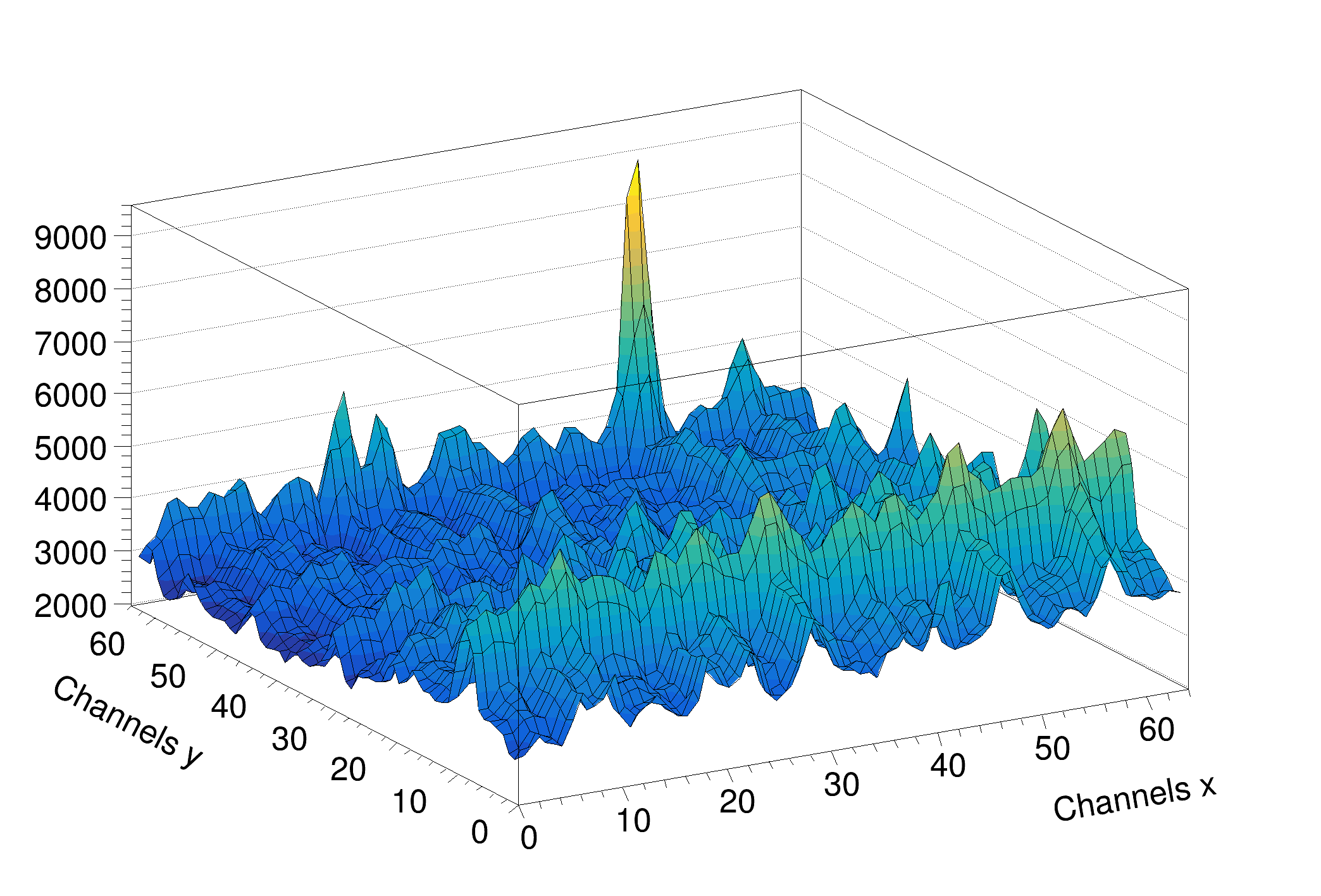### Example 2- Background_gamma256.C

#include <TSpectrum2.h>
void Background_gamma256() {
Int_t i, j;
const Int_t nbinsx = 64;
const Int_t nbinsy = 64;
Double_t xmax = (Double_t)nbinsx;
Double_t ymax = (Double_t)nbinsy;
Double_t** source = new Double_t*[nbinsx];
for (i=0;i<nbinsx;i++)
source[i]=new Double_t[nbinsy];
TString dir = gROOT->GetTutorialDir();
TString file = dir+"/spectrum/TSpectrum2.root";
TFile *f = new TFile(file.Data());
auto back = (TH2F*) f->Get("back2");
auto *s = new TSpectrum2();
for (i = 0; i < nbinsx; i++){
for (j = 0; j < nbinsy; j++){
source[i][j] = back->GetBinContent(i + 1,j + 1);
}
}
for (i = 0; i < nbinsx; i++){
for (j = 0; j < nbinsy; j++)
back->SetBinContent(i + 1,j + 1, source[i][j]);
}
back->Draw("SURF2");
}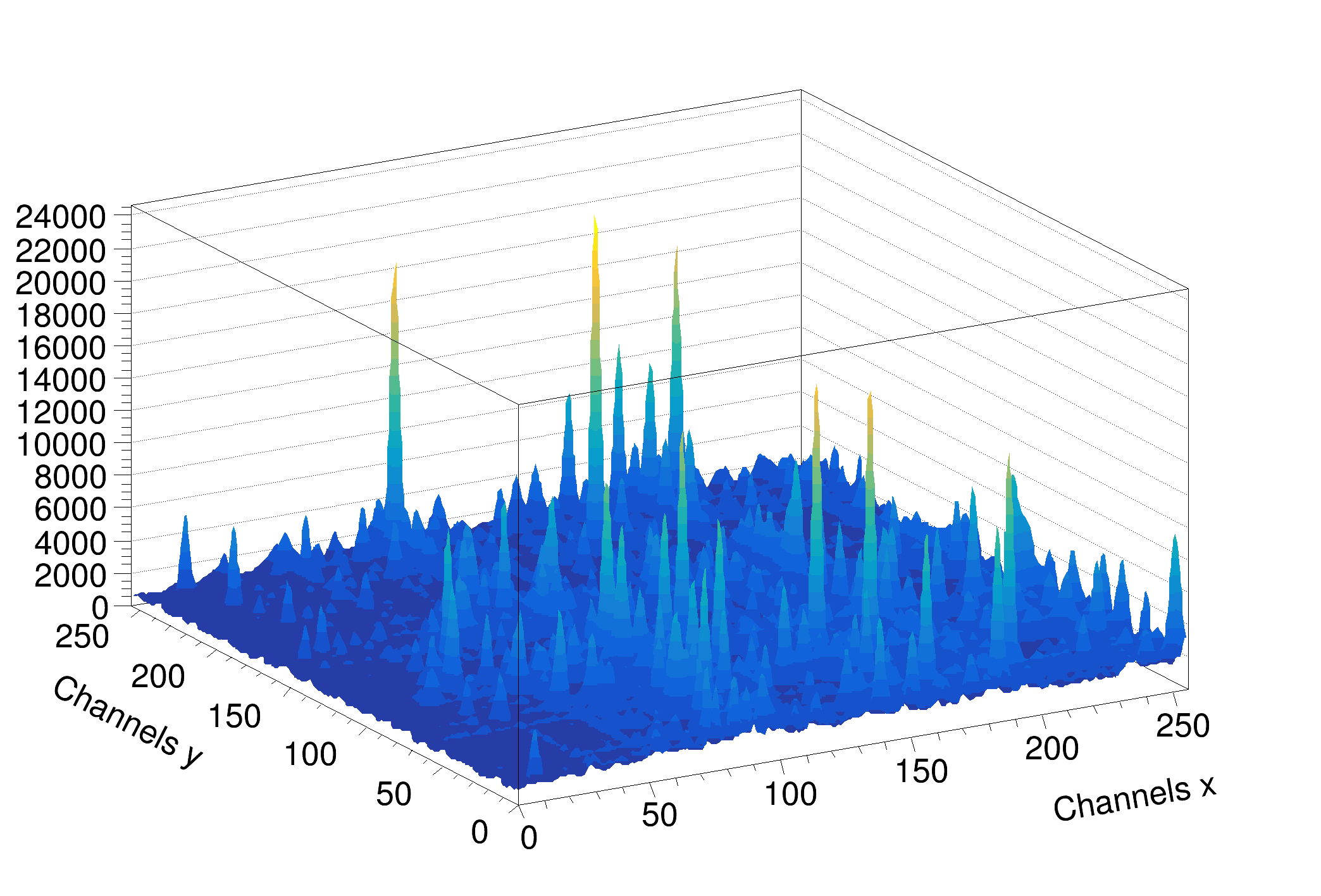### Example 3- Background_synt256.C

#include <TSpectrum2.h>
void Background_synt256() {
Int_t i, j;
const Int_t nbinsx = 64;
const Int_t nbinsy = 64;
Double_t xmax = (Double_t)nbinsx;
Double_t ymax = (Double_t)nbinsy;
Double_t** source = new Double_t*[nbinsx];
for (i=0;i<nbinsx;i++)
source[i]=new Double_t[nbinsy];
TString dir = gROOT->GetTutorialDir();
TString file = dir+"/spectrum/TSpectrum2.root";
TFile *f = new TFile(file.Data());
auto back = (TH2F*) f->Get("back3");
auto *s = new TSpectrum2();
for (i = 0; i < nbinsx; i++){
for (j = 0; j < nbinsy; j++){
source[i][j] = back->GetBinContent(i + 1,j + 1);
}
}
s->Background(source,nbinsx,nbinsy,8,8,
for (i = 0; i < nbinsx; i++){
for (j = 0; j < nbinsy; j++)
back->SetBinContent(i + 1,j + 1, source[i][j]);
}
back->Draw("SURF2");
}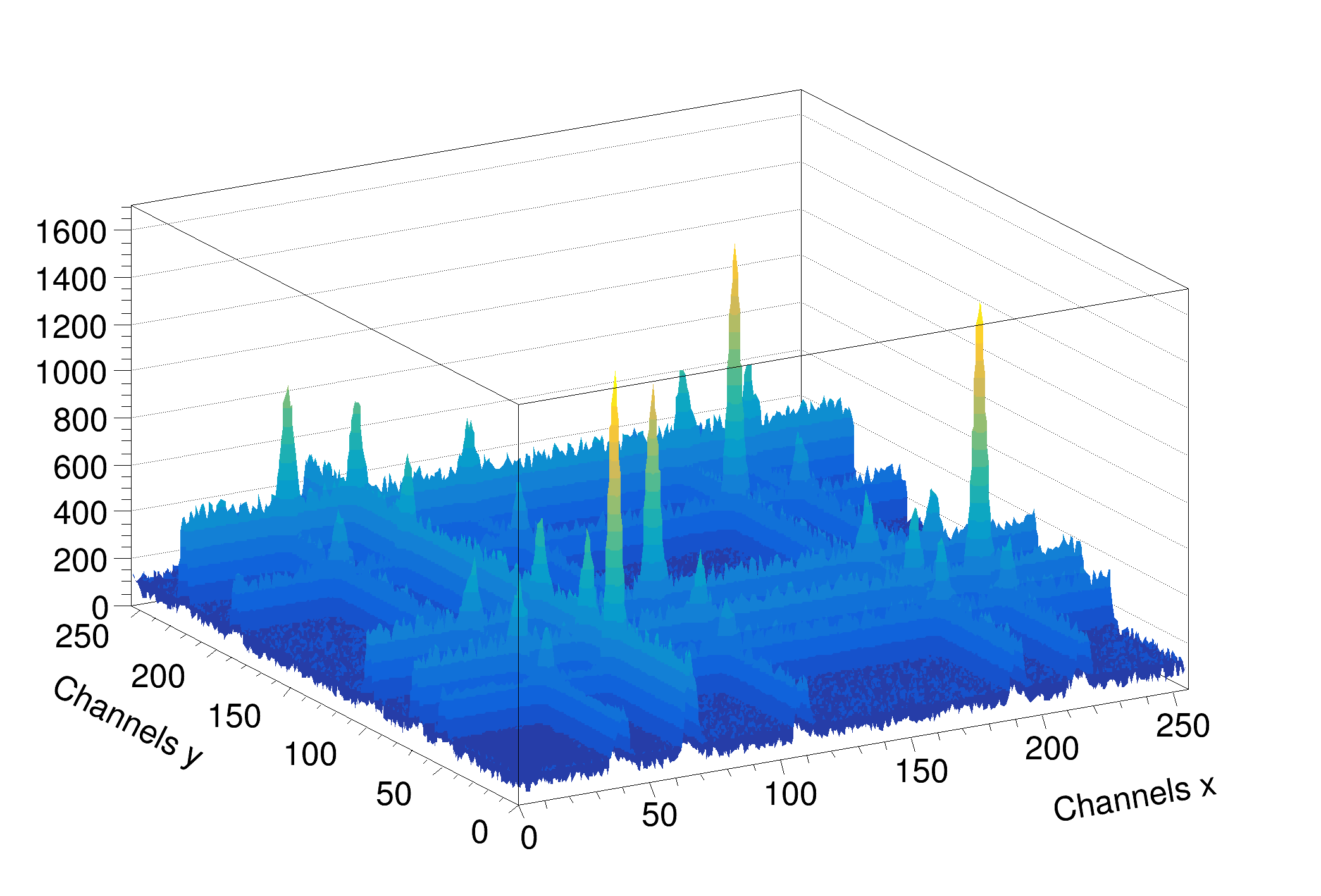Definition at line 367 of file TSpectrum2.cxx.

## ◆ Deconvolution()

 const char * TSpectrum2::Deconvolution ( Double_t ** source, Double_t ** resp, Int_t ssizex, Int_t ssizey, Int_t numberIterations, Int_t numberRepetitions, Double_t boost )

This function calculates deconvolution from source spectrum according to response spectrum The result is placed in the matrix pointed by source pointer.

Function parameters:

• source-pointer to the matrix of source spectrum
• resp-pointer to the matrix of response spectrum
• ssizex-x length of source and response spectra
• ssizey-y length of source and response spectra
• numberIterations, for details we refer to manual
• numberRepetitions, for details we refer to manual
• boost, boosting factor, for details we refer to manual

### Deconvolution

Goal: Improvement of the resolution in spectra, decomposition of multiplets

Mathematical formulation of the 2-dimensional convolution system is

$y(i_1,i_2) = \sum_{k_1=0}^{N_1-1} \sum_{k_2=0}^{N_2-1} h(i_1-k_1,i_2-k_2)x(k_1,k_2)$

$i_1 = 0,1,2, ... ,N_1-1, i_2 = 0,1,2, ... ,N_2-1$

where h(i,j) is the impulse response function, x, y are input and output matrices, respectively, $$N_1, N_2$$ are the lengths of x and h matrices

• let us assume that we know the response and the output matrices (spectra) of the above given system.
• the deconvolution represents solution of the overdetermined system of linear equations, i.e., the calculation of the matrix x.
• from numerical stability point of view the operation of deconvolution is extremely critical (ill-posed problem) as well as time consuming operation.
• the Gold deconvolution algorithm proves to work very well even for 2-dimensional systems. Generalisation of the algorithm for 2-dimensional systems was presented in , .
• for Gold deconvolution algorithm as well as for boosted deconvolution algorithm we refer also to TSpectrum

#### References:

 M. Morhac;, J. Kliman, V. Matouoek, M. Veselsky, I. Turzo.: Efficient one- and two-dimensional Gold deconvolution and its application to gamma-ray spectra decomposition. NIM, A401 (1997) 385-408.

 Morhac; M., Matouoek V., Kliman J., Efficient algorithm of multidimensional deconvolution and its application to nuclear data processing, Digital Signal Processing 13 (2003) 144.

### Example 5 - Deconvolution2_1.c

#include <TSpectrum2.h>
void Deconvolution2_1() {
Int_t i, j;
const Int_t nbinsx = 256;
const Int_t nbinsy = 256;
Double_t xmax = (Double_t)nbinsx;
Double_t ymax = (Double_t)nbinsy;
Double_t** source = new Double_t*[nbinsx];
for (i=0;i<nbinsx;i++)
source[i]=new Double_t[nbinsy];
TString dir = gROOT->GetTutorialDir();
TString file = dir+"/spectrum/TSpectrum2.root";
TFile *f = new TFile(file.Data());
auto decon = (TH2F*) f->Get("decon1");
Double_t** response = new Double_t*[nbinsx];
for (i=0;i<nbinsx;i++)
response[i]=new Double_t[nbinsy];
auto resp = (TH2F*) f->Get("resp1");
auto *s = new TSpectrum2();
for (i = 0; i < nbinsx; i++){
for (j = 0; j < nbinsy; j++){
source[i][j] = decon->GetBinContent(i + 1,j + 1);
}
}
for (i = 0; i < nbinsx; i++){
for (j = 0; j < nbinsy; j++){
response[i][j] = resp->GetBinContent(i + 1,j + 1);
}
}
s->Deconvolution(source,response,nbinsx,nbinsy,1000,1,1);
for (i = 0; i < nbinsx; i++){
for (j = 0; j < nbinsy; j++)
decon->SetBinContent(i + 1,j + 1, source[i][j]);
}
decon->Draw("SURF2");
}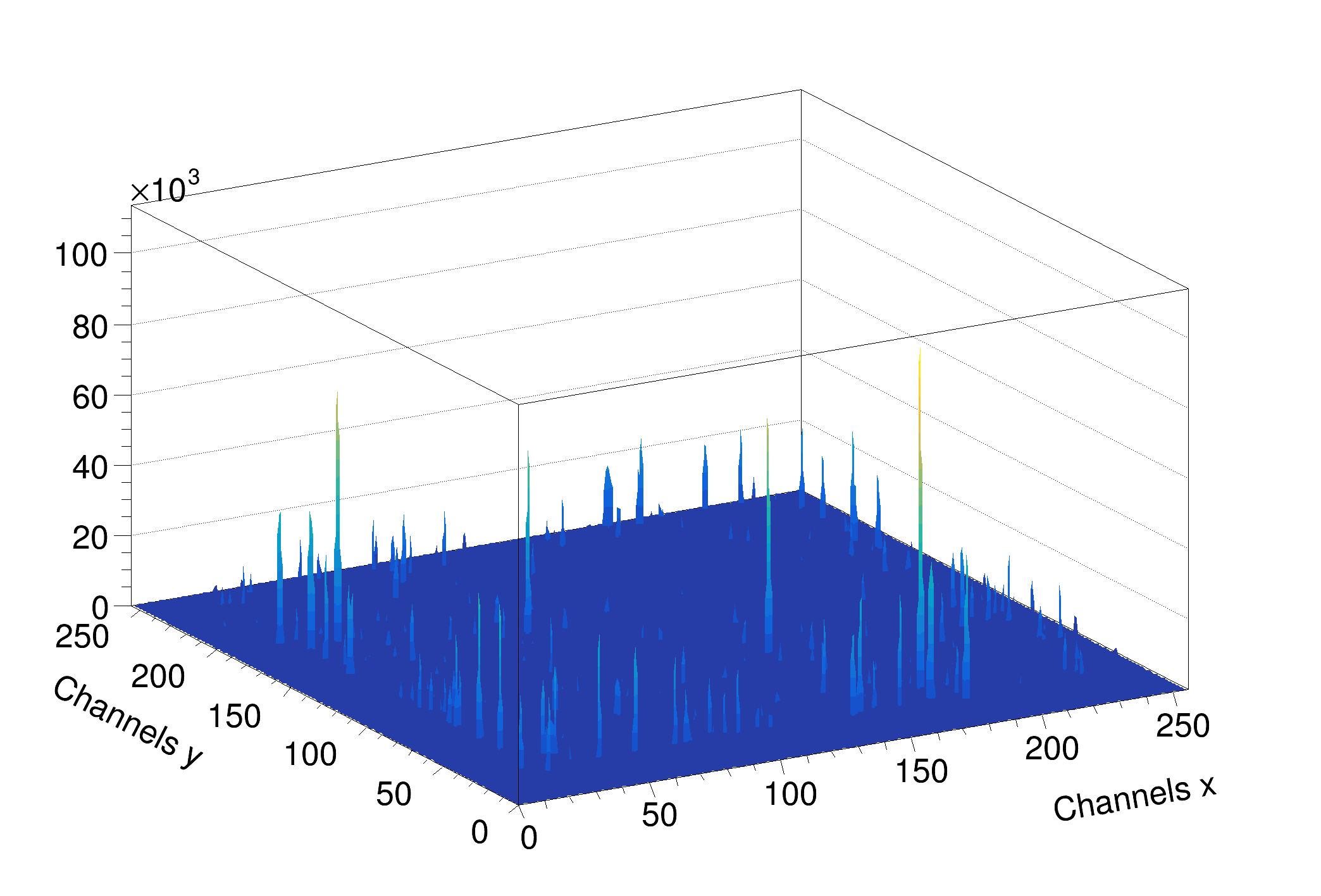### Example 6 - Deconvolution2_2.C

#include <TSpectrum2.h>
void Deconvolution2_2() {
Int_t i, j;
const Int_t nbinsx = 64;
const Int_t nbinsy = 64;
Double_t xmax = (Double_t)nbinsx;
Double_t ymax = (Double_t)nbinsy;
Double_t** source = new Double_t*[nbinsx];
for (i=0;i<nbinsx;i++)
source[i]=new Double_t[nbinsy];
TString dir = gROOT->GetTutorialDir();
TString file = dir+"/spectrum/TSpectrum2.root";
TFile *f = new TFile(file.Data());
auto decon=(TH2F*) f->Get("decon2");
Double_t** response = new Double_t*[nbinsx];
for (i=0;i<nbinsx;i++)
response[i]=new Double_t[nbinsy];
auto resp=(TH2F*) f->Get("resp2");
auto *s = new TSpectrum2();
for (i = 0; i < nbinsx; i++){
for (j = 0; j < nbinsy; j++){
source[i][j] = decon->GetBinContent(i + 1,j + 1);
}
}
for (i = 0; i < nbinsx; i++){
for (j = 0; j < nbinsy; j++){
response[i][j] = resp->GetBinContent(i + 1,j + 1);
}
}
s->Deconvolution(source,response,nbinsx,nbinsy,1000,1,1);
for (i = 0; i < nbinsx; i++){
for (j = 0; j < nbinsy; j++)
decon->SetBinContent(i + 1,j + 1, source[i][j]);
}
decon->Draw("SURF2");
}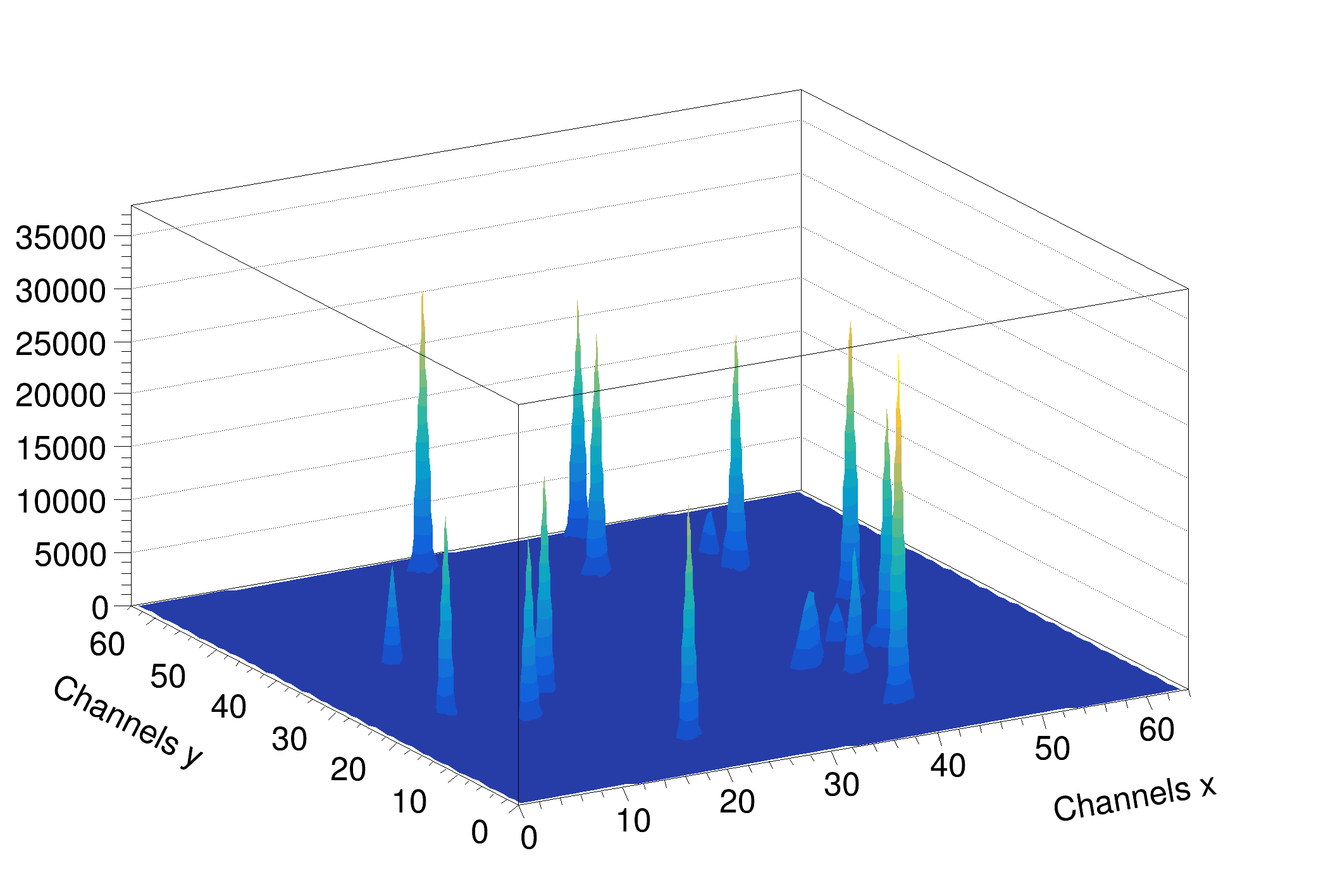### Example 7 - Deconvolution2_HR.C

#include <TSpectrum2.h>
void Deconvolution2_HR() {
Int_t i, j;
const Int_t nbinsx = 64;
const Int_t nbinsy = 64;
Double_t xmax = (Double_t)nbinsx;
Double_t ymax = (Double_t)nbinsy;
Double_t** source = new Double_t*[nbinsx];
for (i=0;i<nbinsx;i++)
source[i]=new Double_t[nbinsy];
TString dir = gROOT->GetTutorialDir();
TString file = dir+"/spectrum/TSpectrum2.root";
TFile *f = new TFile(file.Data());
auto decon = (TH2F*) f->Get("decon2");
Double_t** response = new Double_t*[nbinsx];
for (i=0;i<nbinsx;i++)
response[i]=new Double_t[nbinsy];
auto resp=(TH2F*) f->Get("resp2");
auto *s = new TSpectrum2();
for (i = 0; i < nbinsx; i++){
for (j = 0; j < nbinsy; j++){
source[i][j] = decon->GetBinContent(i + 1,j + 1);
}
}
for (i = 0; i < nbinsx; i++){
for (j = 0; j < nbinsy; j++){
response[i][j] = resp->GetBinContent(i + 1,j + 1);
}
}
s->Deconvolution(source,response,nbinsx,nbinsy,1000,1,1);
for (i = 0; i < nbinsx; i++){
for (j = 0; j < nbinsy; j++)
decon->SetBinContent(i + 1,j + 1, source[i][j]);
}
decon->Draw("SURF2");
}Definition at line 833 of file TSpectrum2.cxx.

## ◆ GetHistogram()

 TH1* TSpectrum2::GetHistogram ( ) const
inline

Definition at line 42 of file TSpectrum2.h.

## ◆ GetNPeaks()

 Int_t TSpectrum2::GetNPeaks ( ) const
inline

Definition at line 43 of file TSpectrum2.h.

## ◆ GetPositionX()

 Double_t* TSpectrum2::GetPositionX ( ) const
inline

Definition at line 44 of file TSpectrum2.h.

## ◆ GetPositionY()

 Double_t* TSpectrum2::GetPositionY ( ) const
inline

Definition at line 45 of file TSpectrum2.h.

## ◆ Print()

 void TSpectrum2::Print ( Option_t * option = "" ) const
virtual

Print the array of positions.

Reimplemented from TNamed.

Definition at line 166 of file TSpectrum2.cxx.

## ◆ Search()

 Int_t TSpectrum2::Search ( const TH1 * hin, Double_t sigma = 2, Option_t * option = "", Double_t threshold = 0.05 )
virtual

This function searches for peaks in source spectrum in hin The number of found peaks and their positions are written into the members fNpeaks and fPositionX.

The search is performed in the current histogram range.

Function parameters:

• hin: pointer to the histogram of source spectrum
• sigma: sigma of searched peaks, for details we refer to manual
• threshold: (default=0.05) peaks with amplitude less than threshold*highest_peak are discarded. 0<threshold<1

By default, the background is removed before deconvolution. Specify the option "nobackground" to not remove the background.

By default the "Markov" chain algorithm is used. Specify the option "noMarkov" to disable this algorithm Note that by default the source spectrum is replaced by a new spectrum

By default a polymarker object is created and added to the list of functions of the histogram. The histogram is drawn with the specified option and the polymarker object drawn on top of the histogram. The polymarker coordinates correspond to the npeaks peaks found in the histogram. A pointer to the polymarker object can be retrieved later via:

TList *functions = hin->GetListOfFunctions();
TPolyMarker *pm = (TPolyMarker*)functions->FindObject("TPolyMarker")

Specify the option "goff" to disable the storage and drawing of the polymarker.

Definition at line 206 of file TSpectrum2.cxx.

## ◆ SearchHighRes()

 Int_t TSpectrum2::SearchHighRes ( Double_t ** source, Double_t ** dest, Int_t ssizex, Int_t ssizey, Double_t sigma, Double_t threshold, Bool_t backgroundRemove, Int_t deconIterations, Bool_t markov, Int_t averWindow )

This function searches for peaks in source spectrum It is based on deconvolution method.

First the background is removed (if desired), then Markov spectrum is calculated (if desired), then the response function is generated according to given sigma and deconvolution is carried out.

Function parameters:

• source-pointer to the matrix of source spectrum
• dest-pointer to the matrix of resulting deconvolved spectrum
• ssizex-x length of source spectrum
• ssizey-y length of source spectrum
• sigma-sigma of searched peaks, for details we refer to manual
• threshold-threshold value in % for selected peaks, peaks with amplitude less than threshold*highest_peak/100 are ignored, see manual
• backgroundRemove-logical variable, set if the removal of background before deconvolution is desired
• deconIterations-number of iterations in deconvolution operation
• markov-logical variable, if it is true, first the source spectrum is replaced by new spectrum calculated using Markov chains method.
• averWindow-averaging window of searched peaks, for details we refer to manual (applies only for Markov method)

### Peaks searching

Goal: to identify automatically the peaks in spectrum with the presence of the continuous background, one-fold coincidences (ridges) and statistical fluctuations - noise.

The common problems connected with correct peak identification in two-dimensional coincidence spectra are

• non-sensitivity to noise, i.e., only statistically relevant peaks should be identified
• non-sensitivity of the algorithm to continuous background
• non-sensitivity to one-fold coincidences (coincidences peak - background in both dimensions) and their crossings
• ability to identify peaks close to the edges of the spectrum region. Usually peak finders fail to detect them
• resolution, decomposition of doublets and multiplets. The algorithm should be able to recognise close positioned peaks.
• ability to identify peaks with different sigma

#### References:

 M.A. Mariscotti: A method for identification of peaks in the presence of background and its application to spectrum analysis. NIM 50 (1967), 309-320.

 M. Morhac;, J. Kliman, V. Matouoek, M. Veselsky, I. Turzo.:Identification of peaks in multidimensional coincidence gamma-ray spectra. NIM, A443 (2000) 108-125.

 Z.K. Silagadze, A new algorithm for automatic photopeak searches. NIM A 376 (1996), 451.

### Examples of peak searching method

SearchHighRes function provides users with the possibility to vary the input parameters and with the access to the output deconvolved data in the destination spectrum. Based on the output data one can tune the parameters.

### Example 8 - Src.C

#include <TSpectrum2.h>
void Src() {
Int_t i, j, nfound;
const Int_t nbinsx = 64;
const Int_t nbinsy = 64;
Double_t xmax = (Double_t)nbinsx;
Double_t ymax = (Double_t)nbinsy;
Double_t** source = new Double_t*[nbinsx];
for (i=0;i<nbinsx;i++)
source[i]=new Double_t[nbinsy];
Double_t** dest = new Double_t*[nbinsx];
for (i=0;i<nbinsx;i++)
dest[i]=new Double_t[nbinsy];
TString dir = gROOT->GetTutorialDir();
TString file = dir+"/spectrum/TSpectrum2.root";
TFile *f = new TFile(file.Data());
auto search = (TH2F*) f->Get("search4");
auto *s = new TSpectrum2();
for (i = 0; i < nbinsx; i++){
for (j = 0; j < nbinsy; j++){
source[i][j] = search->GetBinContent(i + 1,j + 1);
}
}
nfound = s->SearchHighRes(source, dest, nbinsx, nbinsy, 2, 5, kTRUE, 3, kFALSE, 3);
printf("Found %d candidate peaks\n",nfound);
Double_t *PositionX = s->GetPositionX();
Double_t *PositionY = s->GetPositionY();
search->Draw("COL");
auto m = new TMarker();
m->SetMarkerStyle(23);
m->SetMarkerColor(kRed);
for (i=0;i<nfound;i++) {
printf("posx= %d, posy= %d, value=%d\n",(Int_t)(PositionX[i]+0.5), (Int_t)(PositionY[i]+0.5),
(Int_t)source[(Int_t)(PositionX[i]+0.5)][(Int_t)(PositionY[i]+0.5)]);
m->DrawMarker(PositionX[i],PositionY[i]);
}
}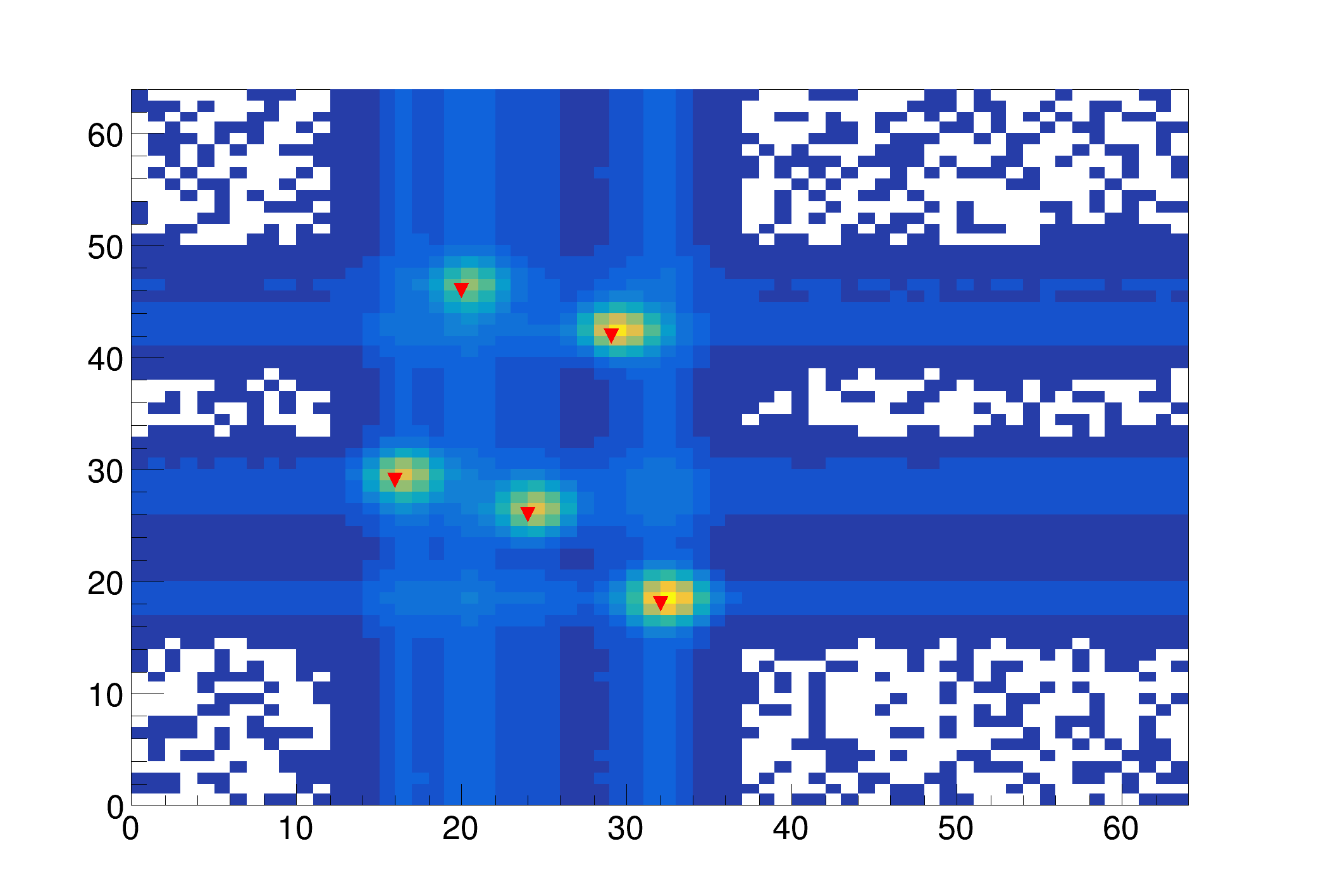### Example 9 - Src2.C

#include <TSpectrum2.h>
void Src2() {
Int_t i, j, nfound;
const Int_t nbinsx = 256;
const Int_t nbinsy = 256;
Double_t xmax = (Double_t)nbinsx;
Double_t ymax = (Double_t)nbinsy;
Double_t** source = new Double_t*[nbinsx];
for (i=0;i<nbinsx;i++)
source[i]=new Double_t[nbinsy];
Double_t** dest = new Double_t*[nbinsx];
for (i=0;i<nbinsx;i++)
dest[i]=new Double_t[nbinsy];
TString dir = gROOT->GetTutorialDir();
TString file = dir+"/spectrum/TSpectrum2.root";
TFile *f = new TFile(file.Data());
auto search = (TH2F*) f->Get("back3");
auto s = new TSpectrum2();
for (i = 0; i < nbinsx; i++){
for (j = 0; j < nbinsy; j++){
source[i][j] = search->GetBinContent(i + 1,j + 1);
}
}
nfound = s->SearchHighRes(source, dest, nbinsx, nbinsy, 2, 10, kTRUE, 10, kFALSE, 3);
printf("Found %d candidate peaks\n",nfound);
Double_t *PositionX = s->GetPositionX();
Double_t *PositionY = s->GetPositionY();
search->Draw("COL");
auto m = new TMarker();
m->SetMarkerStyle(23);
m->SetMarkerColor(kRed);
for (i=0;i<nfound;i++) {
printf("posx= %d, posy= %d, value=%d\n",(Int_t)(PositionX[i]+0.5), (Int_t)(PositionY[i]+0.5),
(Int_t)source[(Int_t)(PositionX[i]+0.5)][(Int_t)(PositionY[i]+0.5)]);
m->DrawMarker(PositionX[i],PositionY[i]);
}
}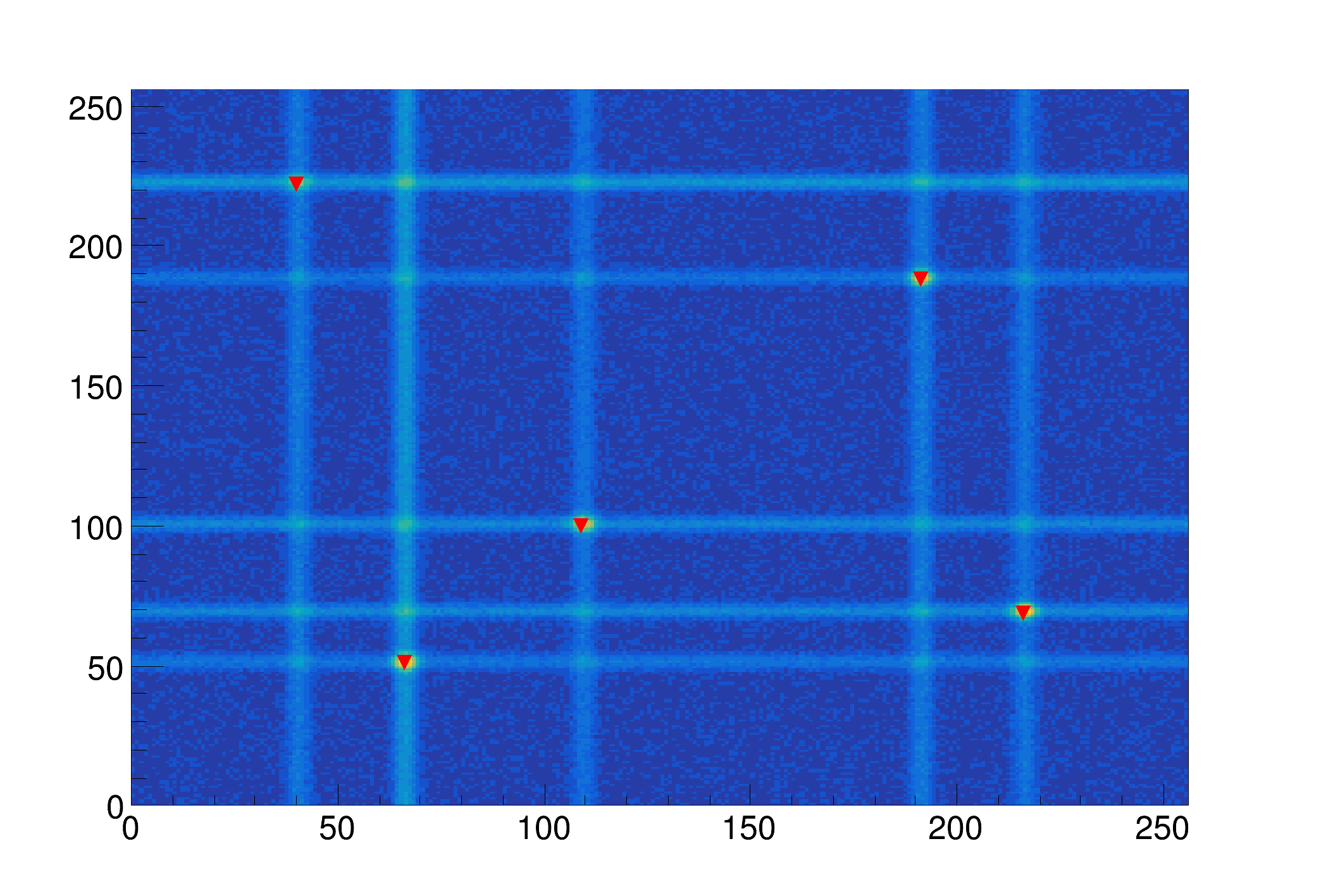### Example 10 - Src3.C

#include <TSpectrum2.h>
void Src3() {
Int_t i, j, nfound;
const Int_t nbinsx = 64;
const Int_t nbinsy = 64;
Double_t xmax = (Double_t)nbinsx;
Double_t ymax = (Double_t)nbinsy;
Double_t** source = new Double_t*[nbinsx];
for (i=0;i<nbinsx;i++)
source[i]=new Double_t[nbinsy];
Double_t** dest = new Double_t*[nbinsx];
for (i=0;i<nbinsx;i++)
dest[i]=new Double_t[nbinsy];
TString dir = gROOT->GetTutorialDir();
TString file = dir+"/spectrum/TSpectrum2.root";
TFile *f = new TFile(file.Data());
auto search = (TH2F*) f->Get("search1");
auto s = new TSpectrum2();
for (i = 0; i < nbinsx; i++){
for (j = 0; j < nbinsy; j++){
source[i][j] = search->GetBinContent(i + 1,j + 1);
}
}
nfound = s->SearchHighRes(source, dest, nbinsx, nbinsy, 2, 2, kFALSE, 3, kFALSE, 1);//3, 10, 100
printf("Found %d candidate peaks\n",nfound);
Double_t *PositionX = s->GetPositionX();
Double_t *PositionY = s->GetPositionY();
search->Draw("CONT");
auto m = new TMarker();
m->SetMarkerStyle(23);
m->SetMarkerColor(kRed);
for (i=0;i<nfound;i++) {
printf("posx= %d, posy= %d, value=%d\n",(Int_t)(PositionX[i]+0.5), (Int_t)(PositionY[i]+0.5),
(Int_t)source[(Int_t)(PositionX[i]+0.5)][(Int_t)(PositionY[i]+0.5)]);
m->DrawMarker(PositionX[i],PositionY[i]);
}
}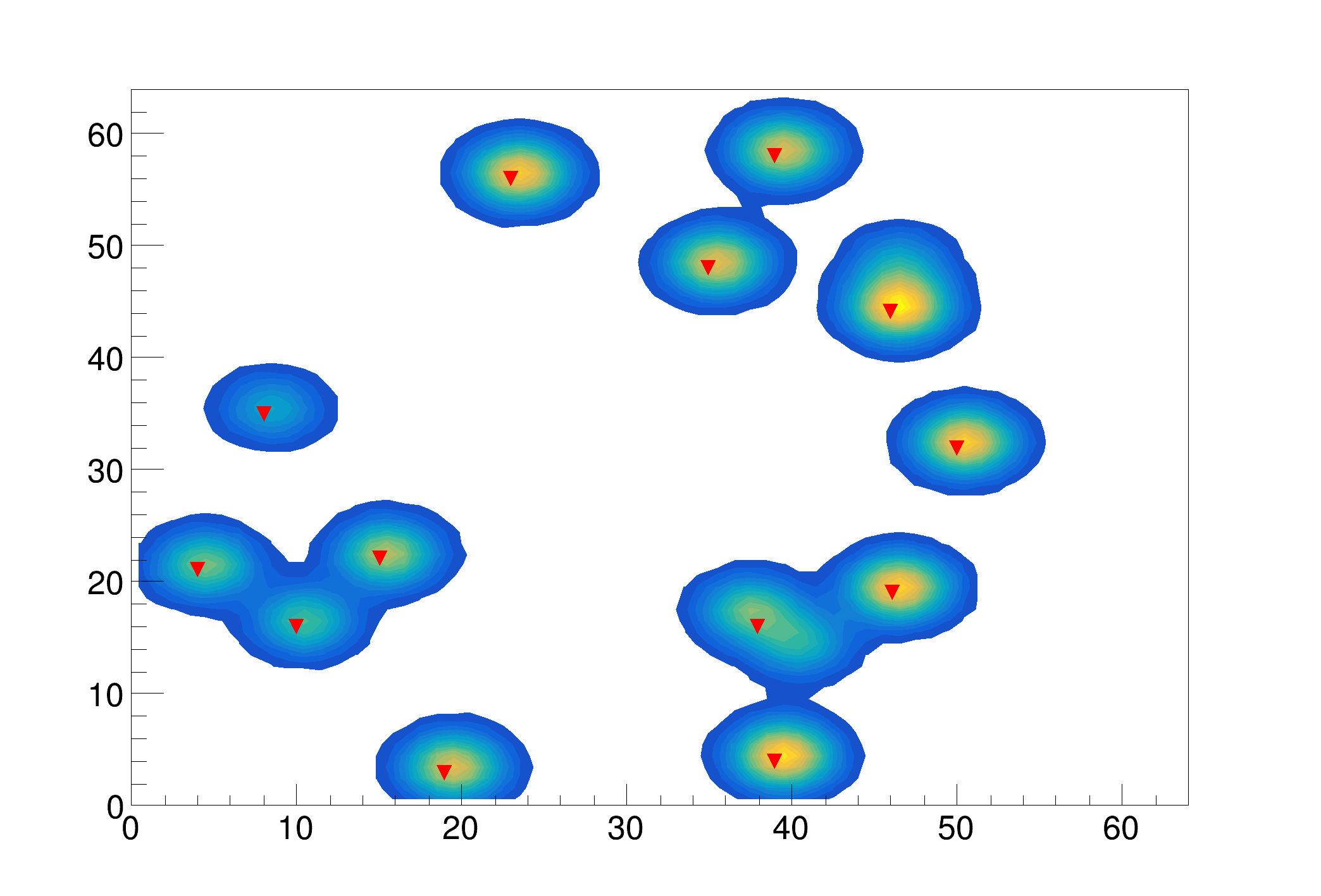### Example 11 - Src4.C

#include <TSpectrum2.h>
void Src4() {
Int_t i, j, nfound;
const Int_t nbinsx = 64;
const Int_t nbinsy = 64;
Double_t xmax = (Double_t)nbinsx;
Double_t ymax = (Double_t)nbinsy;
Double_t** source = new Double_t*[nbinsx];
for (i=0;i<nbinsx;i++)
source[i]=new Double_t[nbinsy];
Double_t** dest = new Double_t*[nbinsx];
for (i=0;i<nbinsx;i++)
dest[i]=new Double_t[nbinsy];
TString dir = gROOT->GetTutorialDir();
TString file = dir+"/spectrum/TSpectrum2.root";
TFile *f = new TFile(file.Data());
auto search = (TH2F*) f->Get("search2");
auto s = new TSpectrum2();
for (i = 0; i < nbinsx; i++){
for (j = 0; j < nbinsy; j++){
source[i][j] = search->GetBinContent(i + 1,j + 1);
}
}
nfound = s->SearchHighRes(source, dest, nbinsx, nbinsy, 3, 5, kFALSE, 10, kTRUE, 3);
printf("Found %d candidate peaks\n",nfound);
Double_t *PositionX = s->GetPositionX();
Double_t *PositionY = s->GetPositionY();
search->Draw("CONT");
auto m = new TMarker();
m->SetMarkerStyle(23);
m->SetMarkerColor(kRed);
for (i=0;i<nfound;i++) {
printf("posx= %d, posy= %d, value=%d\n",(Int_t)(PositionX[i]+0.5), (Int_t)(PositionY[i]+0.5),
(Int_t)source[(Int_t)(PositionX[i]+0.5)][(Int_t)(PositionY[i]+0.5)]);
m->DrawMarker(PositionX[i],PositionY[i]);
}
}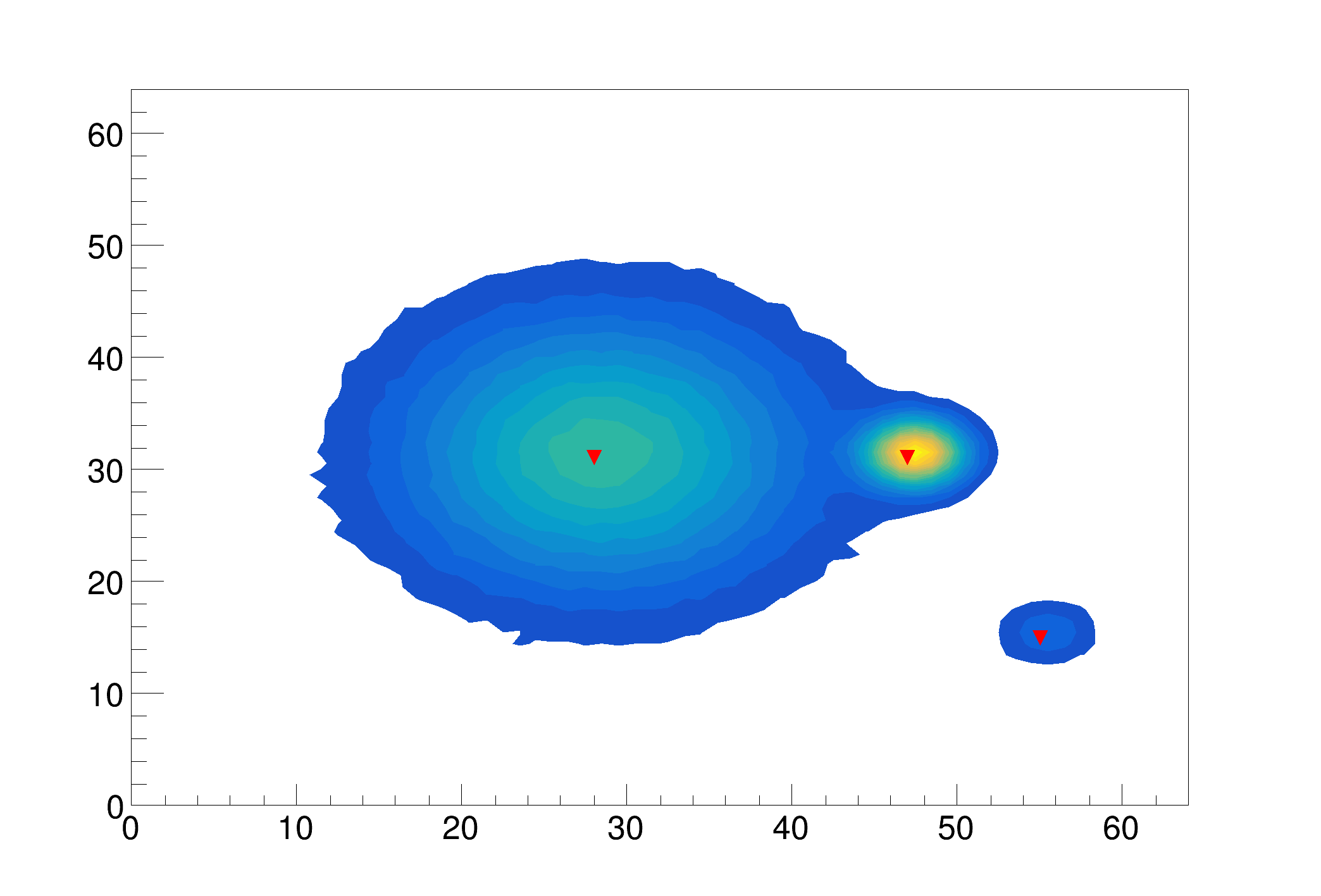### Example 12 - Src5.C

#include <TSpectrum2.h>
void Src5() {
Int_t i, j, nfound;
const Int_t nbinsx = 64;
const Int_t nbinsy = 64;
Double_t xmax = (Double_t)nbinsx;
Double_t ymax = (Double_t)nbinsy;
Double_t** source = new Double_t*[nbinsx];
for (i=0;i<nbinsx;i++)
source[i]=new Double_t[nbinsy];
Double_t** dest = new Double_t*[nbinsx];
for (i=0;i<nbinsx;i++)
dest[i]=new Double_t[nbinsy];
TString dir = gROOT->GetTutorialDir();
TString file = dir+"/spectrum/TSpectrum2.root";
TFile *f = new TFile(file.Data());
auto search = (TH2F*) f->Get("search3;1");
for (i = 0; i < nbinsx; i++){
for (j = 0; j < nbinsy; j++){
source[i][j] = search->GetBinContent(i + 1,j + 1);
}
}
nfound = s->SearchHighRes(source, dest, nbinsx, nbinsy, 2, 5, kFALSE, 10, kFALSE, 1);
printf("Found %d candidate peaks\n",nfound);
Double_t *PositionX = s->GetPositionX();
Double_t *PositionY = s->GetPositionY();
search->Draw("COL");
auto m = new TMarker();
m->SetMarkerStyle(23);
m->SetMarkerColor(kRed);
for (i=0;i<nfound;i++) {
printf("posx= %d, posy= %d, value=%d\n",(Int_t)(PositionX[i]+0.5), (Int_t)(PositionY[i]+0.5),
(Int_t)source[(Int_t)(PositionX[i]+0.5)][(Int_t)(PositionY[i]+0.5)]);
m->DrawMarker(PositionX[i],PositionY[i]);
}
}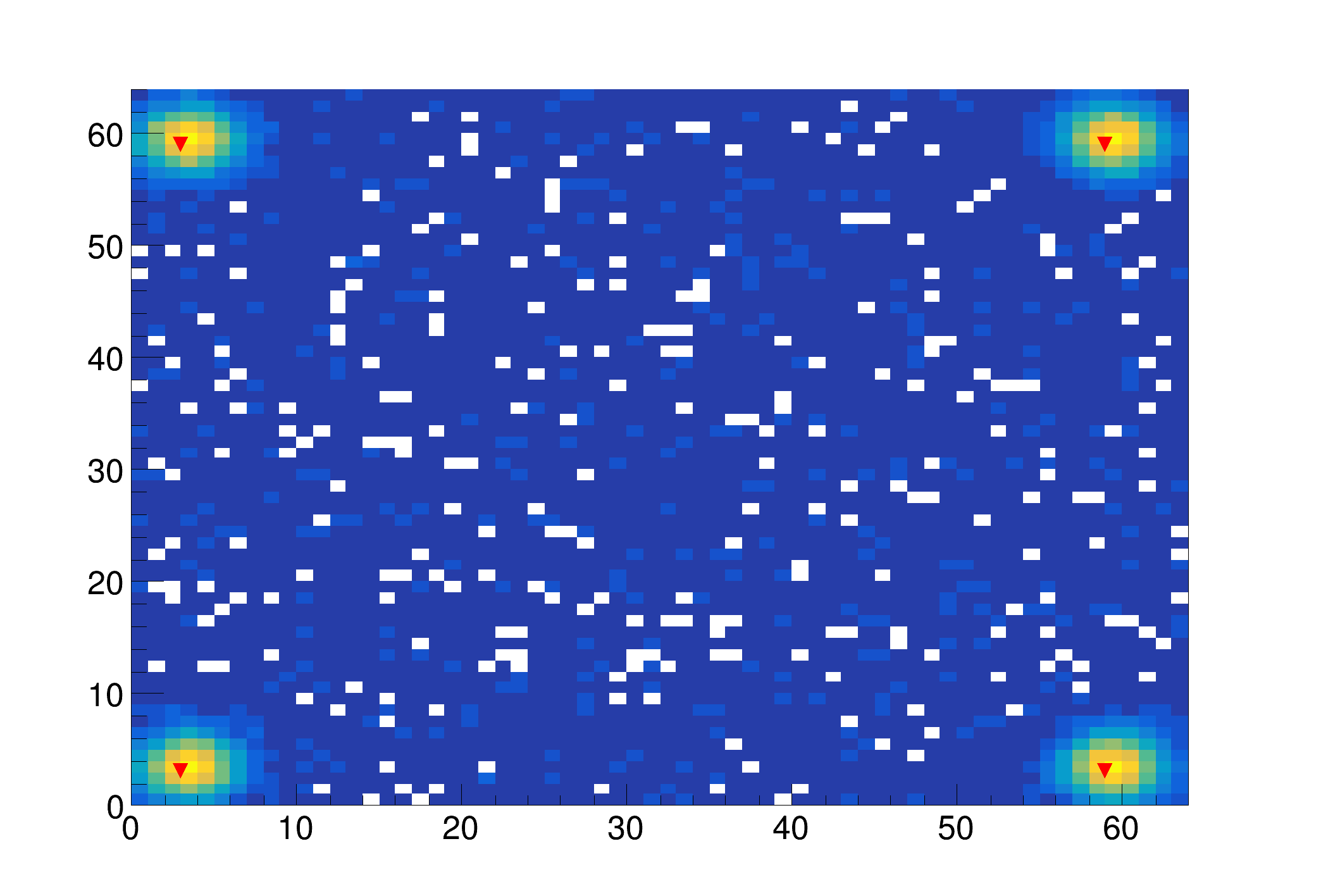Definition at line 1088 of file TSpectrum2.cxx.

## ◆ SetAverageWindow()

 void TSpectrum2::SetAverageWindow ( Int_t w = 3 )
static

static function: Set average window of searched peaks see TSpectrum2::SearchHighRes

Definition at line 104 of file TSpectrum2.cxx.

## ◆ SetDeconIterations()

 void TSpectrum2::SetDeconIterations ( Int_t n = 3 )
static

static function: Set max number of decon iterations in deconvolution operation see TSpectrum2::SearchHighRes

Definition at line 113 of file TSpectrum2.cxx.

## ◆ SetResolution()

 void TSpectrum2::SetResolution ( Double_t resolution = 1 )

NOT USED resolution: determines resolution of the neighboring peaks default value is 1 correspond to 3 sigma distance between peaks.

Higher values allow higher resolution (smaller distance between peaks. May be set later through SetResolution.

Definition at line 287 of file TSpectrum2.cxx.

## ◆ SmoothMarkov()

 const char * TSpectrum2::SmoothMarkov ( Double_t ** source, Int_t ssizex, Int_t ssizey, Int_t averWindow )

This function calculates smoothed spectrum from source spectrum based on Markov chain method.

The result is placed in the array pointed by source pointer.

Function parameters:

• source-pointer to the array of source spectrum
• ssizex-x length of source
• ssizey-y length of source
• averWindow-width of averaging smoothing window

### Smoothing

Goal: Suppression of statistical fluctuations the algorithm is based on discrete Markov chain, which has very simple invariant distribution

$U_2 = \frac{p_{1.2}}{p_{2,1}}U_1, U_3 = \frac{p_{2,3}}{p_{3,2}}U_2 U_1, ... , U_n = \frac{p_{n-1,n}}{p_{n,n-1}}U_{n-1} ... U_2 U_1$

$$U_1$$ being defined from the normalization condition $$\sum_{i=1}^{n} U_i = 1$$ n is the length of the smoothed spectrum and

$p_{i,i\pm1} = A_i \sum_{k=1}^{m} exp\left[\frac{y(i\pm k)-y(i)}{y(i\pm k)+y(i)}\right]$

is the probability of the change of the peak position from channel i to the channel i+1. $$A_i$$ is the normalization constant so that $$p_{i,i-1}+p_{i,i+1}=1$$ and m is a width of smoothing window. We have extended this algorithm to two dimensions.

#### Reference:

 Z.K. Silagadze, A new algorithm for automatic photopeak searches. NIM A 376 (1996), 451.

### Example 4 - Smooth.C

#include <TSpectrum2.h>
void Smooth() {
Int_t i, j;
const Int_t nbinsx = 256;
const Int_t nbinsy = 256;
Double_t xmax = (Double_t)nbinsx;
Double_t ymax = (Double_t)nbinsy;
Double_t** source = new Double_t*[nbinsx];
for (i=0;i<nbinsx;i++)
source[i] = new Double_t[nbinsy];
TString dir = gROOT->GetTutorialDir();
TString file = dir+"/spectrum/TSpectrum2.root";
TFile *f = new TFile(file.Data());
auto smooth = (TH2F*) f->Get("smooth1");
auto *s = new TSpectrum2();
for (i = 0; i < nbinsx; i++){
for (j = 0; j < nbinsy; j++){
source[i][j] = smooth->GetBinContent(i + 1,j + 1);
}
}
s->SmoothMarkov(source,nbinsx,nbinsx,3); //5,7
for (i = 0; i < nbinsx; i++){
for (j = 0; j < nbinsy; j++)
smooth->SetBinContent(i + 1,j + 1, source[i][j]);
}
smooth->Draw("SURF2");
}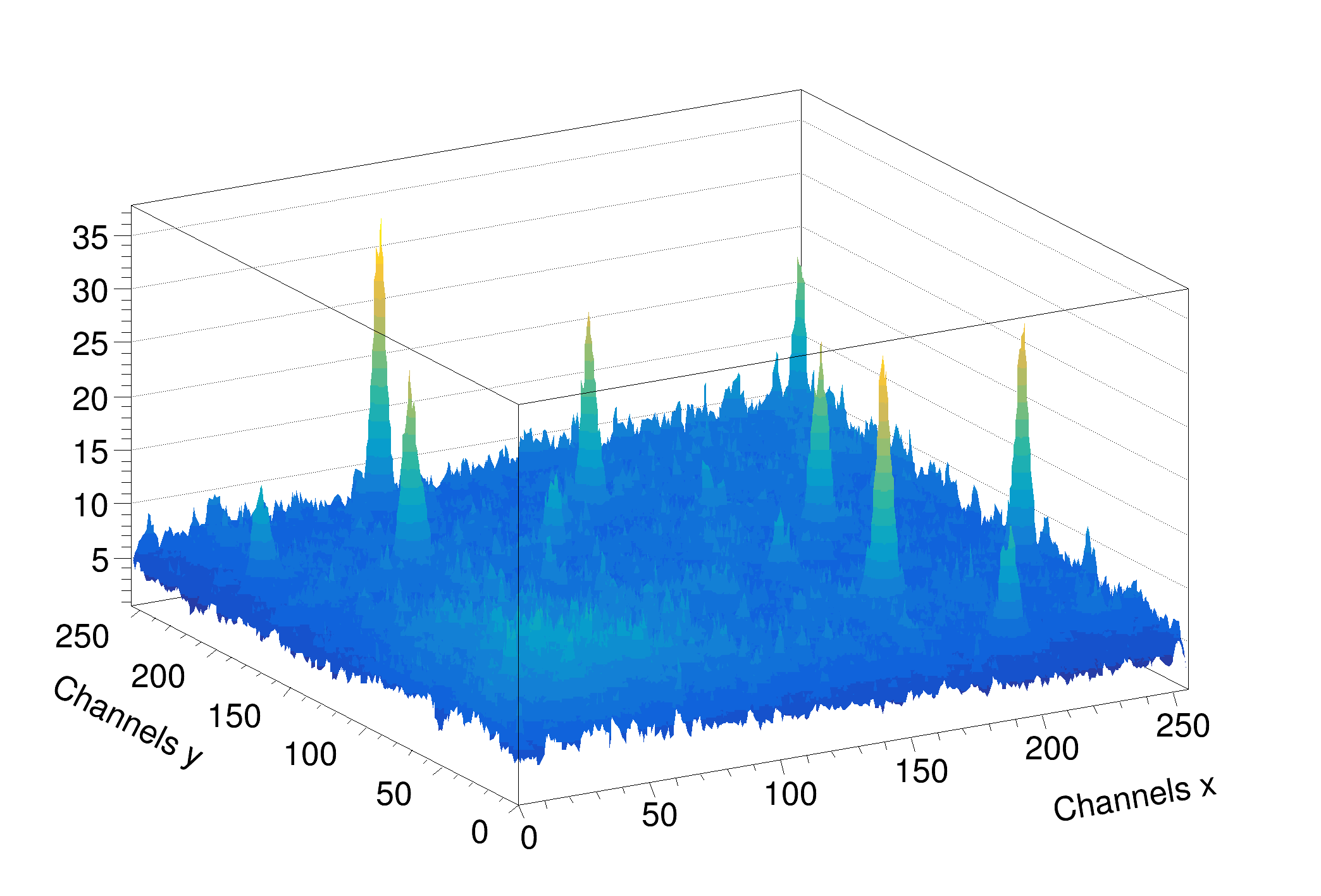Definition at line 580 of file TSpectrum2.cxx.

## ◆ StaticBackground()

 TH1 * TSpectrum2::StaticBackground ( const TH1 * hist, Int_t niter = 20, Option_t * option = "" )
static

static function (called by TH1), interface to TSpectrum2::Background

Definition at line 1719 of file TSpectrum2.cxx.

## ◆ StaticSearch()

 Int_t TSpectrum2::StaticSearch ( const TH1 * hist, Double_t sigma = 2, Option_t * option = "goff", Double_t threshold = 0.05 )
static

static function (called by TH1), interface to TSpectrum2::Search

Definition at line 1710 of file TSpectrum2.cxx.

## ◆ fgAverageWindow

 Int_t TSpectrum2::fgAverageWindow = 3
staticprotected

Average window of searched peaks.

Definition at line 27 of file TSpectrum2.h.

## ◆ fgIterations

 Int_t TSpectrum2::fgIterations = 3
staticprotected

Maximum number of decon iterations (default=3)

Definition at line 28 of file TSpectrum2.h.

## ◆ fHistogram

 TH1* TSpectrum2::fHistogram
protected

resulting histogram

Definition at line 26 of file TSpectrum2.h.

## ◆ fMaxPeaks

 Int_t TSpectrum2::fMaxPeaks
protected

Maximum number of peaks to be found.

Definition at line 20 of file TSpectrum2.h.

## ◆ fNPeaks

 Int_t TSpectrum2::fNPeaks
protected

number of peaks found

Definition at line 21 of file TSpectrum2.h.

## ◆ fPosition

 Double_t* TSpectrum2::fPosition
protected

[fNPeaks] array of current peak positions

Definition at line 22 of file TSpectrum2.h.

## ◆ fPositionX

 Double_t* TSpectrum2::fPositionX
protected

[fNPeaks] X position of peaks

Definition at line 23 of file TSpectrum2.h.

## ◆ fPositionY

 Double_t* TSpectrum2::fPositionY
protected

[fNPeaks] Y position of peaks

Definition at line 24 of file TSpectrum2.h.

## ◆ fResolution

 Double_t TSpectrum2::fResolution
protected

NOT USED resolution of the neighboring peaks

Definition at line 25 of file TSpectrum2.h.

Libraries for TSpectrum2:[legend]

The documentation for this class was generated from the following files: